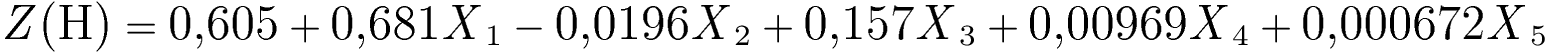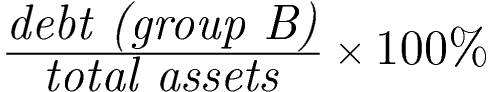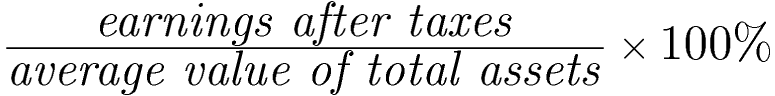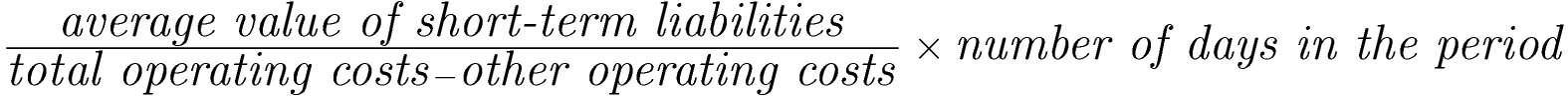Comprehensive software for monitoring and evaluation of the economic situation of the enterprise and early warning# A. Holda's model (2001)

Method of calculationWhere:Model description

A. Holda's model (2001) relies on 5 financial ratios that refer to financial liquidity, indebtedness, efficiency and profitability of operating activity.

Interpretation of the Z(H)-score

• Z (H)= 0 – critical value (bankruptcy threat if less than 0)

• Z (H)≤ 0 -"bankrupt"
• Z (H)> 0 -"non-bankrupt"
• -0,3 ≤ Z(H) ≤ 0,1 -"indifferent" (if Z(H) value is in this interval, then no reliable bankruptcy prediction is possible)
• Model fit (the percentage of correct predictions): 92.5%.
• The higher is the Z(H), the better is the overall financial standing of the company (as the lower is the threat of bankruptcy within the next year of performance).
• The increase of the Z(H) function over time means the improvement of the company's financial situation.
• In the case of the Z(H) below 0 (which signalizes bankruptcy threat) it is recommended to run a detailed analysis of financial performance to identify which aspects require immediate healing procedures.

Detailed decription of respective ratios and their impact on the Z(H)-score value

Constant value +0.605

The constant, free factor results from statistical estimates when building the model, it allows setting the limit value of Z(H) at the level of zero

X1 – current ratio

Method of calculationRatio's weight: +0.681
Units: times

Remarks

The ratio is calculated like the current ratio available in the eanaliza.pl system in the group of liquidity ratios. It uses the data for the current period.

Ratio's interpretation

• A higher value of the ratio means a higher level of liquidity which reduces the risk of bankruptcy.
• In the model, the X1 ratio has the highest weight (with a positive sign), which means the greatest impact of this ratio on the function, therefore a change in the current ratio over time may significantly change the value of the Z (H) function.

X2 – debt to assets ratio

Method of calculationRatio's weight: -0.0196
Units: %

Remarks

The ratio is calculated like the debt ratio available in the eanaliza.pl system in the group of debt management ratios. It uses the data for the current period.

Ratio's interpretation

• A higher value of the ratio means higher indebtedness of the company, which increases the risk of bankruptcy.
• In the model, the ratio X2 has a weight with a negative sign, which means that an increase in the ratio (thus an increase in debt) will lower the value of the function Z(H).

X3 – total assets turnover

Method of calculationRatio's weight: +0.157
Units: %

Remarks

The ratio is partially calculated like the total assets turnover ratio (TAT) available in the eanaliza.pl system in the group of efficiency ratios, except for:

• the numerator includes all revenues (revenues from all activities),
• the denominator includes the average value of total assets: (initial value at the beginning of the period + final value at the end of period) / 2
Warning: the value for the first period (first column in the table) is equal to the total assets from the current period, as the value for the previous period is not available.

Ratio's interpretation

A higher value of the ratio means greater efficiency of the company in generating revenues from its activities, which reduces the risk of bankruptcy.

In the model, the ratio X3 has a weight with a positive sign (at a high level), which means that an increase in the ratio (higher efficiency) will increase the value of the function Z(H).

X4 – return on assets

Method of calculationRatio's weight: +0.00969
Units: %

Remarks

The ratio is partially calculated like the return on assets (ROA), except for:

• the numerator includes the financial result (even if it is negative),
• the denominator includes the average value of total assets: (initial value at the beginning of the period + final value at the end of period) / 2
Warning: the value for the first period (first column in the table) is equal to the total assets from the current period, as the value for the previous period is not available.

Ratio's interpretation

• A higher value of the ratio means greater ability of the company to generate profit based on its resources (assets).
• In the model, the ratio X4 has a weight with a positive sign, which means that the increase in the ratio (increase in profitability) will increase the value of the function Z(H).
• In case of a negative result (financial loss), the ratio X4 will decrease the value of the Z(H) function, increasing the risk of bankruptcy.

X5 – current liabilities turnover ratio (in days)

Method of calculationRatio's weight: +0.000672
Units: days

Remarks

The ratio is built according to the original formula by A. Holda.
To calculate it we take in:

• the numerator the average value of short-term liabilities: (initial value at the beginning of the period + final value at the end of period) / 2,
Warning: the value for the first period (first column in the table) is equal to the total short-term liabilities from the current period, as the value for the previous period is not available.
• the denominator the total cost of activity without other operating costs (the cost of sold goods, materials and products).

Ratio's interpretation

• A higher value of the ratio X5 means a longer turnover period of short-term liabilities, and therefore a longer period of settling short-term liabilities.
• A longer repayment period for short-term liabilities reduces the risk of bankruptcy.
• In the model, the X5 ratio has a weight with a positive sign (at a very low level), which means that its increase will increase the value of the function Z(H).

Based on:
HOLDA A.: Prognozowanie bankructwa jednostki w warunkach gospodarki polskiej z wykorzystaniem funkcji dyskryminacyjnej ZH. Rachunkowość nr 5/2001. (in Polish)

We use cookies to ensure you have the best experience on our website. If you continue to use this site, we will assume that you are happy with it.